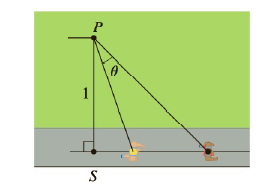Chapter 3.7, Problem 75E

Chapter
Section
Textbook Problem

# An observer stands at a point P, one unit away from a track. Two runners start at the point S in the figure and run along the track. One runner runs three times as fast as the other. Find the maximum value of the observer’s angle of sight θ between the runners. [Hint: Maximize tan θ .]To determine

To find:

The maximum value of the observer’s angle of sight θ between the runners

Explanation

1) Concept:

If f'x<0 then fx is the absolute minimum value

Formula:

tanα+θ=tanα+tanθ1-tanα·tanθ

2) Calculation:

An observer stands at a point P, one unit away from the track

We have to find value of θ

tanα=Side opposite to αSide adjacent to α

=1t1

=t

tanα+θ=Side opposite to α+θSide adjacent to α+θ

tanα+θ=3t1

By using formula,

tanα+tanθ1-tanα·tanθ=3t

Substitute value of tanα

t+tanθ1-t·tanθ=3t

t+tanθ=3t1-t·tanθ

t+tanθ=3t-3t2·tanθ

tanθ+3t2·tanθ=3t-t

1+3t2

### Still sussing out bartleby?

Check out a sample textbook solution.

See a sample solution

#### The Solution to Your Study Problems

Bartleby provides explanations to thousands of textbook problems written by our experts, many with advanced degrees!

Get Started

#### Evaluate the expression sin Exercises 116. (23)2

Finite Mathematics and Applied Calculus (MindTap Course List)

#### Find the mean for the following set of scores: 2, 7, 9, 4, 5, 3, 0, 6

Essentials of Statistics for The Behavioral Sciences (MindTap Course List)

#### The graph of θ = 2 in polar coordinates is a: circle line spiral 3-leaved rose

Study Guide for Stewart's Single Variable Calculus: Early Transcendentals, 8th